Documentation

# balancmr

Balanced model truncation via square root method

## Syntax

```GRED = balancmr(G)
GRED = balancmr(G,order)
[GRED,redinfo] = balancmr(G,key1,value1,...)
[GRED,redinfo] = balancmr(G,order,key1,value1,...)
```

## Description

`balancmr` returns a reduced order model `GRED` of `G` and a struct array `redinfo` containing the error bound of the reduced model and Hankel singular values of the original system.

The error bound is computed based on Hankel singular values of `G`. For a stable system these values indicate the respective state energy of the system. Hence, reduced order can be directly determined by examining the system Hankel singular values, σι.

With only one input argument `G`, the function will show a Hankel singular value plot of the original model and prompt for model order number to reduce.

This method guarantees an error bound on the infinity norm of the additive error `G-GRED` ∥ ∞ for well-conditioned model reduced problems :

`${‖G-Gred‖}_{\infty }\le 2\sum _{k+1}^{n}{\sigma }_{i}$`

This table describes input arguments for `balancmr`.

Argument

Description

`G`

LTI model to be reduced. Without any other inputs, `balancmr` will plot the Hankel singular values of `G` and prompt for reduced order

`ORDER`

(Optional) Integer for the desired order of the reduced model, or optionally a vector packed with desired orders for batch runs

A batch run of a serial of different reduced order models can be generated by specifying `order = x:y`, or a vector of positive integers. By default, all the anti-stable part of a system is kept, because from control stability point of view, getting rid of unstable state(s) is dangerous to model a system.

`'MaxError'` can be specified in the same fashion as an alternative for `'``Order``'`. In this case, reduced order will be determined when the sum of the tails of the Hankel singular values reaches the `'MaxError'`.

This table lists the input arguments `'key'` and its `'value'`.

Argument

Value

Description

`'MaxError'`

Real number or vector of different errors

Reduce to achieve H error. When present, `'``MaxError``'` overrides `ORDER` input.

`'Weights'`

`{Wout,Win}` cell array

Optional 1-by-2 cell array of LTI weights `Wout` (output) and `Win` (input). The weights must be stable, minimum phase and invertible. When you supply these weights, `balancmr` finds the reduced model that minimizes the Hankel norm of

`${W}_{out}^{-1}\left(G-{G}_{red}\right){W}_{in}^{-1}.$`

You can use weighting functions to make the model reduction algorithm focus on frequency bands of interest. See:

As an alternative, you can use `balred` to focus model reduction on a particular frequency band without defining a weighting function. Using `balancmr` and providing your own weighting functions allows more precise control over the error profile.

Default weights are both identity.

`'Display'`

`'on'``'` or `'off'`

Display Hankel singular plots (default `'off'`).

`'Order'`

Integer, vector or cell array

Order of reduced model. Use only if not specified as 2nd argument.

This table describes output arguments.

Argument

Description

`GRED`

LTI reduced order model. Becomes multidimensional array when input is a serial of different model order array

`REDINFO`

A STRUCT array with three fields:

• `REDINFO.ErrorBound` (bound on ∥ G-GRED ∥∞)

• `REDINFO.StabSV` (Hankel SV of stable part of G)

• `REDINFO.UnstabSV` (Hankel SV of unstable part of G)

`G` can be stable or unstable, continuous or discrete.

## Examples

collapse all

If you do not specify any target order for the reduced model, `balancmr` displays the Hankel singular values of the model and prompts you to choose a reduced-model order.

For this example, use a random 30th-order state-space model.

```rng(1234,'twister'); % fix random seed for example repeatability G = rss(30,5,4); G1 = balancmr(G)```
`Please enter the desired order: (>=0)`

Examine the Hankel singular value plot.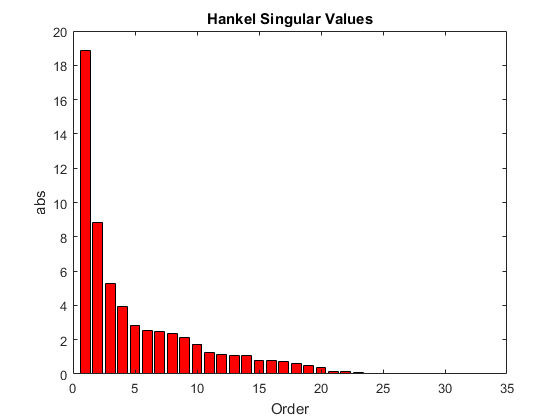The plot shows that most of the energy of the system can be captured in a 20th-order approximation. In the command window, enter 20. `balancmr` returns `G1`.

Examine the response of the original and reduced models.

`sigma(G,G1)`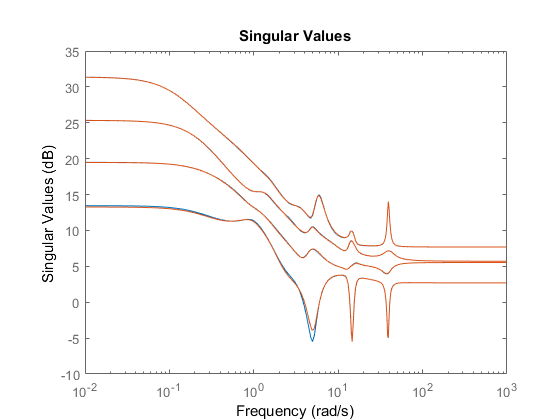The 20th-order approximation matches the dynamics of the original 30th-order model fairly well.

When you have particular target order or orders in mind, you can use balancmr to reduce a high-order model to those orders. For this example, use a random 30th-order state-space model.

```rng(1234,'twister'); % fix random seed for example repeatability G = rss(30,5,4);```

Use a scalar input argument to reduce the model to a single order. For example, compute a 20th-order approximation.

```[G1,info1] = balancmr(G,20); sigma(G,G1)```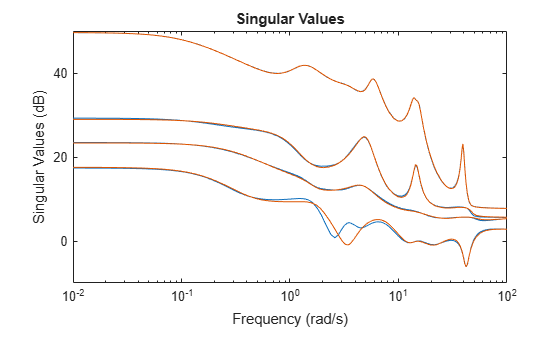Use a vector to generate several approximations. The following command returns an array of models of even orders from 10 to 18.

```[G2,info2] = balancmr(G,[10:2:18]); sigma(G,G2)```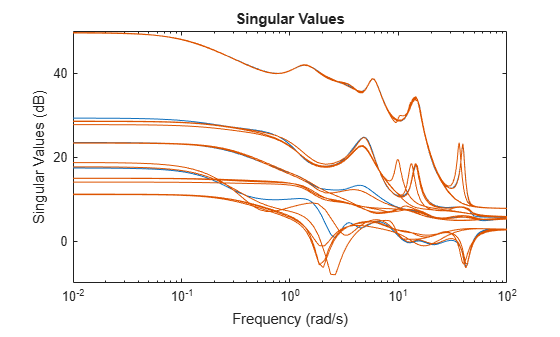Obtain the lowest-order approximation such that the sum of the Hankel singular values of the truncated states does not exceed a specified value. For this example, use a random 30th-order state-space model.

```rng(1234,'twister'); % fix random seed for example repeatability G = rss(30,5,4);```

Compute two approximate models, one for which the error does not exceed 0.1, and a second for which the error does not exceed 0.5. To do so, provide these values in an array. `balancmr` returns an array of approximate models.

```Gr = balancmr(G,'MaxError',[0.1 0.5]); size(Gr)```
```2x1 array of state-space models. Each model has 5 outputs, 4 inputs, and between 24 and 26 states. ```

Examine the results.

`sigma(G,Gr)`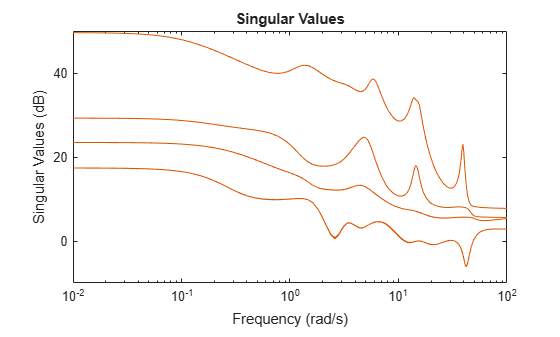Reduce a 4th-order system to a second-order approximation with emphasis on the frequency band 10 rad/s - 100 rad/s. Consider the following system.

```sys = tf(1,[1 0.5 1]) + tf(100*[1/10 1],[1 10 1000]); bode(sys)```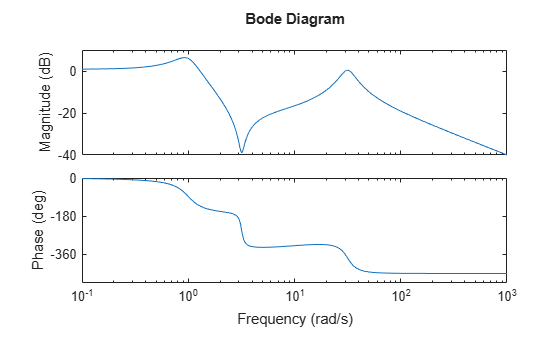To focus the model-reduction algorithm on the higher-frequency dynamics, specify a function with a bandpass profile.

```s = tf('s'); w1 = (s+1)/(s/10+1)/(s/60+1)*(s/600+1); bodemag(w1)```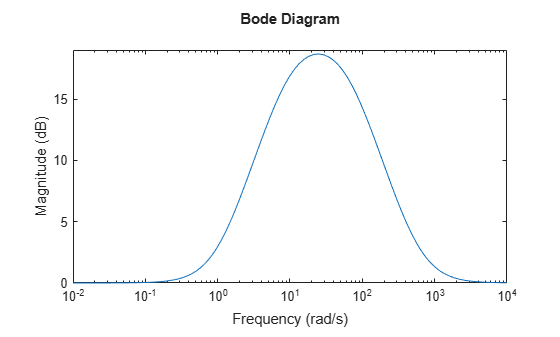The plot confirms that the weighting function `w1` has the desired profile, peaking between 10 rad/s and 100 rad/s. To perform the reduction, specify the inverse of this profile as the output weight, using the `'Weights'` option of `balancmr`.

```weight = {1/w1,1}; wrsys = balancmr(sys,2,'Weights',weight);```

Compare the result with a second-order model obtained without the weighting.

```rsys = balancmr(sys,2); bode(sys,rsys,wrsys) legend('Original','Unweighted','Weighted')```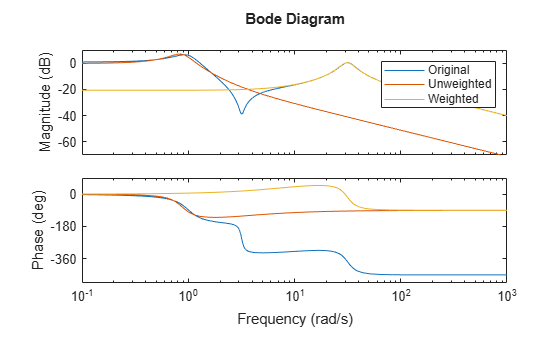The model obtained with the weighting function provides a better match for the dynamics in the frequency band 10 rad/s - 100 rad/s.

Use a weighting function to control the frequency dependence of the error between the original and reduced models.

For this example, load a 48-state SISO model and reduce it to 6th order.

```load(fullfile(matlabroot,'examples','robust','balancmrData.mat'),'bplant') bplant6 = balancmr(bplant,6); bode(bplant,bplant6,bplant-bplant6,logspace(0,2,200)) legend('Original','Reduced','Error')```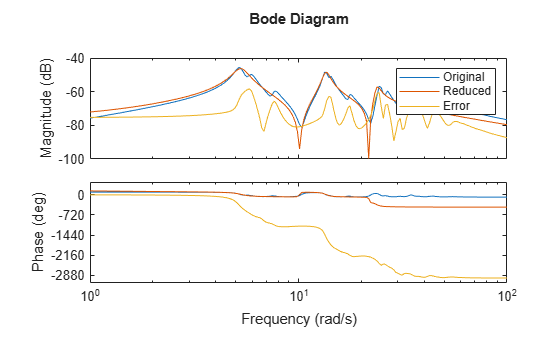The error is fairly uniformly distributed in frequency. Create a weighting function that allows for a larger error at frequencies below 100 rad/s. In this case, use a biproper function that has unit gain at low frequency, but drops to -40 dB at higher frequencies.

```W = tf(0.01*[1 1.4e2 1e4],[1 14 100]); bodemag(W,bplant)```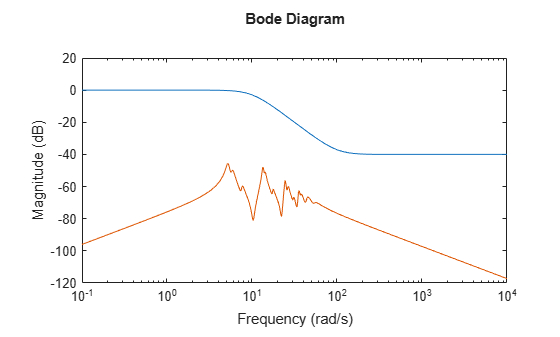Reduce the model to 6th order again, using this weighting function. Because `bplant` is a SISO model, you can use the function as either input or output weight.

```bplant6W = balancmr(bplant,6,'Weights',{W,1}); bode(bplant,bplant6W,bplant-bplant6W,logspace(0,2,200)) legend('Original','Reduced w/Weight','Error')```The error is now larger at low frequencies, with a correspondingly better match at high frequencies between the original and reduced models.

## Algorithms

Given a state space (A,B,C,D) of a system and k, the desired reduced order, the following steps will produce a similarity transformation to truncate the original state-space system to the kth order reduced model.

1. Find the SVD of the controllability and observability grammians

P = Up Σp VpT

Q = UqΣq VqT

2. Find the square root of the grammians (left/right eigenvectors)

Lp = Up Σp½

Lo = Uq Σq½

3. Find the SVD of (LoTLp)

LoT Lp = U Σ VT

4. Then the left and right transformation for the final kth order reduced model is

SL,BIG = Lo U(:,1:k) Σ(1;k,1:k))–½

SR,BIG = Lp V(:,1:k) Σ(1;k,1:k))–½

5. Finally,

`$\left[\begin{array}{cc}\stackrel{^}{A}& \stackrel{^}{B}\\ \stackrel{^}{C}& \stackrel{^}{D}\end{array}\right]=\left[\begin{array}{cc}{S}_{L,BIG}^{T}A{S}_{R,BIG}& {S}_{L,BIG}^{T}B\\ C{S}_{R,BIG}& D\end{array}\right]$`

The proof of the square root balance truncation algorithm can be found in .

## References

 Glover, K., “All Optimal Hankel Norm Approximation of Linear Multivariable Systems, and Their Lµ-error Bounds,“ Int. J. Control, Vol. 39, No. 6, 1984, p. 1145-1193

 Safonov, M.G., and R.Y. Chiang, “A Schur Method for Balanced Model Reduction,” IEEE Trans. on Automat. Contr., Vol. 34, No. 7, July 1989, p. 729-733

## See Also

#### Introduced before R2006a

##### Support평가판 신청